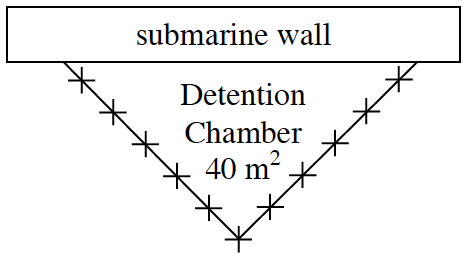### Home > APCALC > Chapter 5 > Lesson 5.3.2 > Problem5-127

5-127.YELLOW SUBMARINE, Part Three

Ring O’s new three-chamber detention chamber was so successful that his friend John Lemon is jealous. Not to be outdone, John decides to make his chamber in the shape of an isosceles triangle. His chamber will still have $40$ square meters of area. What dimensions should the chamber have so that Mr. Lemon can buy the least amount of barbed wire?

$\text{Since you know the area of the detention chamber }=\frac{1}{2}(\text{base})(\text{height})=40.$

Solve for $b$ or $h$.

Dissect the isosceles triangle into two right triangles, label its dimensions in terms of $b$ or $h$. Then use the the Pythagorean Theorem to solve for the hypotenuse.

Write a perimeter function. Then use Calculus to optimize it.